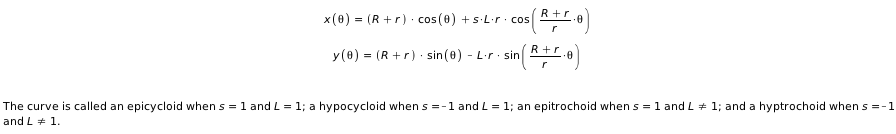Since their inception in Maple 15, the Math Apps in Maple have given students and teachers the ability to explore and illustrate a wide variety of mathematical and scientific concepts. These fun and easy to use educational demonstrations are designed to illustrate various mathematical and physical concepts.

Each demonstration has a brief section describing the concepts involved and interactive features that bring the concepts to life and allow students to test their understanding. Students can type mathematical equations, click buttons, adjust sliders, draw graphs, and more. With Maple's powerful engine updating the calculations in the background, the students will see the result of their actions instantly.

In Maple 16, there are over 100 new Math Apps ranging in scope from precalculus and calculus to statistics and physics! See the full list of Math Apps in Maple 16.Example: Drawing the Graph of a Quadratic Function

The following example allows the student to learn how to draw the graph of a quadratic equation (a parabola).

Generate a quadratic function and try to draw it on the graph. Use the radio buttons below the graph to graph different elements in different colors. When you are finished, click the corresponding check boxes to see how accurate you were.

Example: Epicycloids and Hypocycloids
This next app allows the student to user sliders, buttons and checkboxes to adjust the parameters of an epicycloid, hypocycloid, epitrochoid or hypotrochoid, the equations for which are given by:Example: The Monkey and the Coconut

The following classic thought experiment, The Monkey and the Coconut is used to illustrate the effect of the gravitational force on a projectile.

A coconut tree is growing in the middle of a small river. Two monkeys arrive at the bank and notice a small ripe coconut about to fall into the river. In order to get the coconut, the monkeys make a plan: On the count of three, the first monkey will shake the tree, while the other will jump across the river and catch the coconut as it falls, landing on the far bank.

How should the second monkey aim her jump in order to catch the coconut?

Adjust the monkey's initial speed and jump angle so that she catches the coconut.

Note: Air resistance is negligible, and time has been slowed down.

Monte Carlo Approximation of π

This example from statistics shows how to use the probabilistic Monte Carlo method to estimate the value of π:

1. A number of points are selected at random within a square with an inscribed circle
2. The number of points inside the circle divided by the total number of points will result in an approximation of π/4. We multiply by 4 to get the estimate for π.

Adjust the number of points being plotted and see how it affects the approximation of Pi.How to Proceed: Pricing & Purchase Evaluate Upgrade Get Price Quote Buy Online